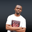Related Tags

php
join
implode
communitycreator

# How to convert an array to a string in PHPNDUKWE CHIDERA K.

A smooth interplay between different types of variables in PHP can pop up when we write code, which we can achieve with arrays and strings.

### The implode() and join() functions

These two functions are built-in functions in PHP. You can use them to format your array contents into a string. This new string variable still holds the type of an array.

However, these functions are not entirely different functions of their own. join() is an alias of the implode() function. Therefore, implode() can be replaced with join() in any usage, and vice versa.

### Syntax

implode(string $separator, array$nameOfArray)


To use join(), just change implode() to join(), and the rest remains the same.

### Explanation

• First, we call the implode function.
• The function takes two parameters.
• The first parameter is the separator of the array items to be formatted into a string in a double/single quote. Common separators are space (""), comma (","), underscore ("_"), the plus sign ("+"), pipe sign (|), and so on.
• The second parameter is the name of the array.

Note: You can omit the first parameter, but the content of the string will be lumped together as one word.

### Code

<?php
$index = array('human','high','jump'); //printing array print_r($index);

//using implode() and join() to join array items
$fine = implode("+",$index);
$mine = join($index);

print "imploded array with + as separator: $fine\n"; print "joined array with no separator parameter:$mine\n";

?>
A few examples

### Explanation

In the code above, we print an array in line 5. In lines 8 and 9, we use the implode() and join() methods to combine array elements. Then, in lines 11 and 12, we return the new strings.

RELATED TAGS

php
join
implode
communitycreator

CONTRIBUTORNDUKWE CHIDERA K.
RELATED COURSES

View all Courses

Keep Exploring

Learn in-demand tech skills in half the time